# Probability Theory Fundamentals

### Introduction

In this series I want to explore some introductory concepts from statistics that may occur helpful for those learning machine learning or refreshing their knowledge. Those topics lie at the heart of data science and arise regularly on a rich and diverse set of topics. It is always good to go through the basics again — this way we may discover new knowledge which was previously hidden from us, so let’s go on.

The first part will introduce fundamentals of probability theory.

### Probability

Why do we need probabilities when we already have such a great mathematical tooling? We have calculus to work with functions on the infinitesimal scale and to measure how they change. We developed algebra to solve equations, and we have dozens of other areas of mathematics that help us to tackle almost any kind of hard problem we can think of.

The difficult part is that we all live in a chaotic universe where things can’t be measured exactly most of the time. When we study real world processes we want to learn about numerous random events that distort our experiments. Uncertainty is everywhere and we must tame it to be used for our needs. That is when probability theory and statistics come into play.

Nowadays those disciplines lie in the center of artificial intelligence, particle physics, social science, bio-informatics and in our everyday lives.

If we are getting to talk about statistics, it is better to settle on what is a probability. Actually, this question has no single best answer. We will go through various views on probability theory below.

### Frequentist probabilities

Imagine we were given a coin and want to check whether it is fair or not. How do we approach this? Let’s try to conduct some experiments and record 1 if heads come up and 0 if we see tails. Repeat this 1000 tosses and count each 0 and 1. After we had some tedious time experimenting, we got those results: 600 heads (1s) and 400 tails (0s). If we then count how frequent heads or tails came up in the past, we will get 60% and 40% respectively. Those frequencies can be interpreted as probabilities of a coin coming up heads or tails. This is called a frequentist view on the probabilities.

### Conditional probabilities

Frequently we want to know the probability of an event given some other event has occurred. We write conditional probability of an event A given event B as P(A | B). Take rains for example:

• What is the probability of a rain given we see thunder
• What is the probability of a rain given it is sunny?
• 打雷时下雨的概率有多大？
• 晴天下雨的概率有多大？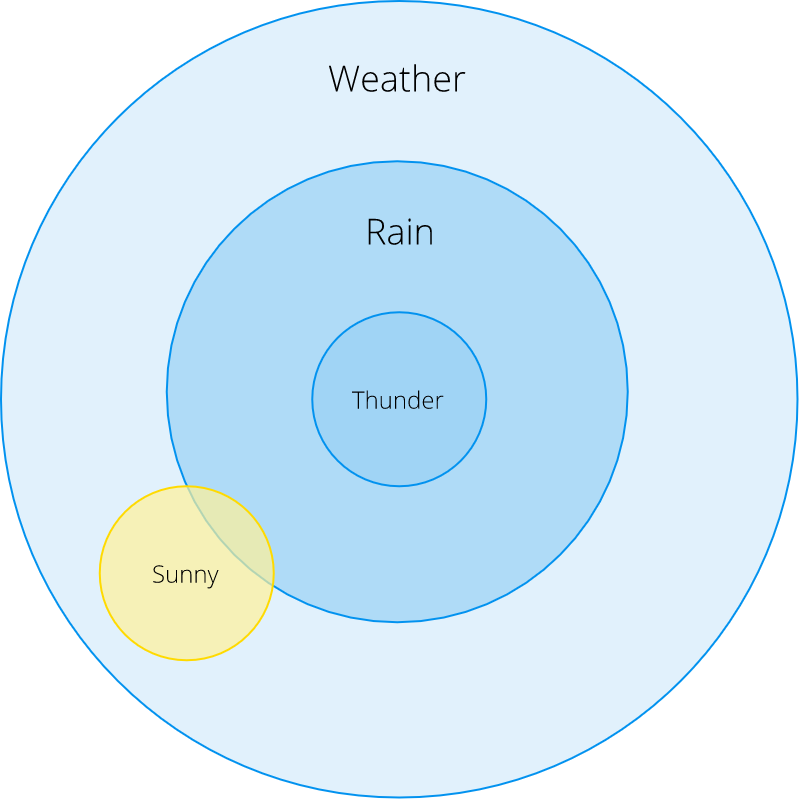From this Euler diagram we can see that P(Rain | Thunder) = 1: there is always raining when we see thunder (yes, it is not exactly true, but we’ll take this as true in our example).

What about P(Rain | Sunny)? Visually this probability is quite small but how can we formulate this mathematically and do exact calculations? Conditional probability is defined as:

P（Rain | Sunny）是多少呢？ 直觉上这个概率很小，但是我们怎样才能在数学上做出这个准确的计算呢？ 条件概率定义为：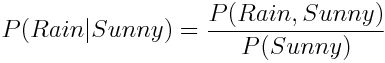In words, we divide probability of both Rain and Sunny by the probability of a Sunny weather.

### Dependent and independent events

Events are called independent if the probability of one event does not influence the other in any way. Take for example the probability of rolling a dice and getting a 2 for the first time and for the second time. Those events are independent. We can state this as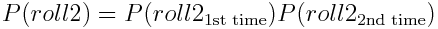But why this formula works? First, let’s rename events for 1st and 2nd tosses as A and B to remove notational clutter and then rewrite probability of a roll explicitly as joint probability of both rolls we had seen so far: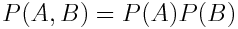And now multiply and divide P(A) by P(B) (nothing changes, it can be cancelled out) and recall the definition of conditional probability: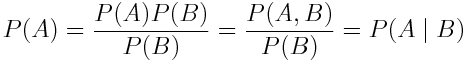If we read expression above from right to left we find that P(A | B) = P(A). Basically, this means that A is independent of B! The same argument goes for P(B) and we are done.

### Bayesian view on probability

There is an alternative way to look at probabilities called Bayesian. Frequentist approach to statistics supposes the existence of one best concrete combination of model parameters we are looking to find. On the other hand, Bayesian way treats parameters in a probabilistic manner and views them as random variables. In Bayesian statistics, each parameter has its own probability distribution which tells us how probable are parameters given the data. Mathematically this can be written as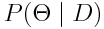It all starts with a simple theorem that allows us to compute conditional probabilities based on prior knowledge: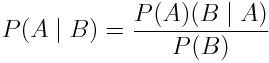Despite its simplicity, Bayes Theorem has an immense value, vast area of application and even special branch of statistics called Bayesian statistics. There is a very nice blog post about Bayes Theorem if you are interested in how it can be derived — it is not that hard at all.

### Distributions

What is a probability distribution anyways? It is a law that tells us probabilities of different possible outcomes in some experiment formulated as a mathematical function. As each function, a distribution may have some parameters to adjust its behavior.

When we measured relative frequencies of a coin toss event we have actually calculated a so-called empirical probability distribution. It turns out that many uncertain processes in our world can be formulated in terms of probability distributions. For example, our coin outcomes have a Bernoulli distribution and if we wanted to calculate a probability of heads after n trials we may use a Binomial distribution.

It is convenient to introduce a concept analogous to a variable that may be used in probabilistic environments — a random variable. Each random variable has some distribution assigned to it. Radom variables are written in upper case by convention, and we may use ~ symbol to specify a distribution assigned to a variable.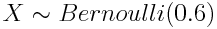This means that random variable X is distributed according to a Bernoulli distribution with probability of success (heads) equal to 0.6.

### Continuous and discrete probability distributions

Probability distributions can come in two flavors: — Discrete ones are dealing with random variables that have a finite countable number of values, as it was the case with coins and Bernoulli distribution. Discrete distributions are defined by functions called Probability Mass Functions (PMF) — Continuous distributions deal with continuous random variables that can (in theory) have an infinite number of values. Think of velocity and acceleration measured with noisy sensors. Continuous distributions are defined by functions called Probability Density Functions (PDF)

Those types of distributions differ in mathematical treatment: you typically will use summations with discrete and integrals with continuous probability distributions. Take expected value for an example: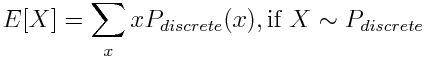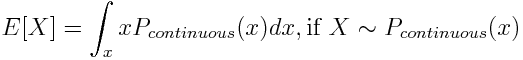### Samples and statistics

Suppose we are doing research on human height and are eager to publish a mind-blowing scientific paper. We measured the height of some strangers on the street, therefore our measurements are independent. A process when we select a random subset of data from the true population is called sampling. Statistic is the funcion used to summarize the data from using values from the sample. The statistic you likely met before is the sample mean: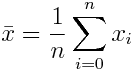Another example is sample variance: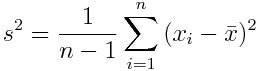This formula captures in overall how all data points differ from their mean.

### What if I want more?

You want to go in-depth with probability theory and statistics? Great! You will definitely benefit from this knowledge whether you are want to get a solid understanding of the theory behind machine learning or just curious.

• Entry level: Khan Academy is a great free resource. The course will get you through the basics in a very intuitive and simple form
• Intermediate level: All of the statistics by Larry Wasserman is a great and concise resource that will present you almost all of the important topics in statistics. Beware that this book assumes you are familiar with linear algebra and calculus